• 2018-06-19 14:00:05

# 二叉树的非递归遍历

• 二叉树的三种遍历方式也可以通过非递归的方法借助栈来实现。
• 通过控制节点的出栈和入栈先后顺序来实现对树的不同方式的遍历。

## 非递归先根遍历二叉树

• 当栈不为空或者当前节点为不为空，执行操作：
• 从根节点开始，依次访问树中最左端的节点并入栈，当节点为空停止入栈。
• 取栈顶元素为当前节点并出栈，如果当前节点有右子树，则遍历其右子树。
void PreorderPrint(SearchBTree T)       // 先序根(序)遍历二叉树并打印出结点值
{
if (T == nullptr)
{
cout << "Empty tree!";
exit(1);
}
Stack S = new StackNode;        // 借助栈来实现
initStack(S);

TreeNode* pT = T;       // 创建临时结点指向根节点

while (pT || !isEmpty(S))           // 当结点不为空或者栈不为空执行循环
{
if (pT)                         // 当pT不为空
{
Push(S, pT);                // pT入栈
cout << pT->data << " ";    // 打印当前结点
pT = pT->left;              // pT移动指向其左孩子
}
else
{       // pT为空，则
pT = getTop(S);
Pop(S);                  // 若pT为空表示左子树的左孩子全部遍历完，依次出栈
pT = pT->right;             // 当左孩子及根结点遍历完之后，开始遍历其右子树
}
}
cout << endl;
delete S;
}

## 非递归中根遍历二叉树

• 当栈不为空或者当前节点为不为空，执行操作：
• 同样地，先依次将根节点及其左子树最左端的节点入栈，但不进行访问。当节点为空，则停止入栈。
• 访问栈顶元素作为当前节点并出栈，如果当前节点有右子树，则遍历访问其右子树。
void InorderPrint(SearchBTree T)        // 中根(序)遍历二叉树并打印出结点值
{
if (T == nullptr)
{
cout << "Empty tree!";
exit(1);
}
Stack S = new StackNode;        // 借助栈来实现
initStack(S);

TreeNode* pT = T;       // 创建临时结点指向根节点

while (pT || !isEmpty(S))           // 当结点不为空或者栈不为空执行循环
{
if (pT)                         // 当pT不为空
{
Push(S, pT);                // pT入栈
pT = pT->left;              // pT移动指向其左孩子
}
else
{       // pT为空，则
pT = getTop(S);
Pop(S); cout << pT->data << " ";    // 若pT为空表示左子树的左孩子全部遍历完，依次出栈并打印
pT = pT->right;             // 当左孩子及根结点遍历完之后，开始遍历其右子树
}
}
cout << endl;
delete S;
}

## 非递归后根遍历二叉树

• 非递归后根遍历相比前两个有点麻烦，需要引入一个中间变量标记已经访问节点。
• 当栈不为空或者当前节点为不为空，执行操作：
• 依次将根节点及其左子树的左端节点入栈，但不进行访问，当节点为空，停止入栈。
• 取栈顶元素作为当前节点，如果当前节点的右孩子（右子树）不为空且其右孩子不是上一次访问的节点。则当前节点变为其右子树，遍历其右子树。如果当前节点的右孩子为空或者其右孩子已经被访问，则访问当前节点，标记当前节点为已访问节点，出栈。将当前节点置为空（此时右孩子访问过了），继续取栈顶元素（为了访问根节点）。
void PostorderPrint(SearchBTree T)      // 先根(序)遍历二叉树并打印出结点值
{
if (T == nullptr)
{
cout << "Empty tree!";
exit(1);
}
Stack S = new StackNode;        // 借助栈来实现
initStack(S);

TreeNode* pT = T;       // 创建临时结点指向根节点
TreeNode* qT = nullptr;

while (pT || !isEmpty(S))           // 当结点不为空或者栈不为空执行循环
{
if (pT)                         // 当pT不为空
{
Push(S, pT);                // pT入栈
pT = pT->left;              // pT移动指向其左孩子
}
else
{
pT = getTop(S);         // 取栈顶元素作为当前结点
if (pT->right && pT->right != qT)   // 若当前节点有右孩子且不是上一次已经被访问的结点
{
pT = pT->right;     // 指向其右孩子
}
else
{                       // 若当前结点没有右孩子或者未被访问，则
Pop(S);             // 出栈
cout << pT->data << " ";    // 访问当前结点的数据
qT = pT;                    // 令pT记录当前结点，用于稍后判断是否已经被访问过
pT = nullptr;               // 将当前结点赋值为空
}
// 当左孩子及根结点遍历完之后，开始遍历其右子树
}
}
cout << endl;
delete S;
}

## 附总的代码实现

#include<iostream>
using namespace std;
typedef int ElemType;

typedef struct _TreeNode {  // 定义二叉查找树的结构
ElemType data;          // 数据
struct _TreeNode* left;         // 指向左孩子指针
struct _TreeNode* right;        // 指向其右孩子指针
}TreeNode, *SearchBTree;

typedef SearchBTree StackElemType;
typedef struct StackNode {          // 定义栈的结构（基于链表）
SearchBTree data;
StackNode *next;
}*Stack;

void initStack(Stack St)        // 初始化栈，把头节点看作是栈顶
{
St->next = nullptr;
}

int isEmpty(Stack St)
{
return St->next == nullptr;
}

void Push(Stack St, SearchBTree x)      // 每次从将元素插入到头节点的位置，头节点上移
{
StackNode* q = new StackNode;
q->data = x;
q->next = St->next;     // q的next域指向St(头节点)的后继结点
St->next = q;           // St(头节点/栈顶)next域指向q，实现栈往上移
}

void Pop(Stack St)      // 出栈
{
StackNode* p = St->next;        // 临时结点指向头结点(栈顶)的后继结点
St->next = p->next;     // 栈顶下移
delete p;           // 释放空间
}

SearchBTree getTop(Stack St)
{
return St->next->data;
}

SearchBTree EmptyTree(SearchBTree T)    // 初始化、构造一颗空树/销毁一颗树
{
if (!T)
{
EmptyTree(T->left);     // 递归地释放空间
EmptyTree(T->right);
delete T;
}
return nullptr;
}

void Insert(SearchBTree &T, ElemType x)
{
if (!T)
{
TreeNode* pT = new TreeNode;        // 申请节点空间
pT->data = x;                       // 为节点赋值
pT->left = pT->right = nullptr;
T = pT;                             // 将pT赋给T
}
else
{
if (x < T->data)                // 如果x小于某个结点的数据
Insert(T->left, x);         // 递归地在其左子树上寻找空结点插入
else if (x > T->data)           // 如果x大于某个结点的数据
Insert(T->right, x);        // 递归地在其左子树上寻找空结点插入
}
}

void PreorderPrint(SearchBTree T)       // 先序根(序)遍历二叉树并打印出结点值
{
if (T == nullptr)
{
cout << "Empty tree!";
exit(1);
}
Stack S = new StackNode;        // 借助栈来实现
initStack(S);

TreeNode* pT = T;       // 创建临时结点指向根节点

while (pT || !isEmpty(S))           // 当结点不为空或者栈不为空执行循环
{
if (pT)                         // 当pT不为空
{
Push(S, pT);                // pT入栈
cout << pT->data << " ";    // 打印当前结点
pT = pT->left;              // pT移动指向其左孩子
}
else
{       // pT为空，则
pT = getTop(S);
Pop(S);                  // 若pT为空表示左子树的左孩子全部遍历完，依次出栈
pT = pT->right;             // 当左孩子及根结点遍历完之后，开始遍历其右子树
}
}
cout << endl;
delete S;
}

void InorderPrint(SearchBTree T)        // 中根(序)遍历二叉树并打印出结点值
{
if (T == nullptr)
{
cout << "Empty tree!";
exit(1);
}
Stack S = new StackNode;        // 借助栈来实现
initStack(S);

TreeNode* pT = T;       // 创建临时结点指向根节点

while (pT || !isEmpty(S))           // 当结点不为空或者栈不为空执行循环
{
if (pT)                         // 当pT不为空
{
Push(S, pT);                // pT入栈
pT = pT->left;              // pT移动指向其左孩子
}
else
{       // pT为空，则
pT = getTop(S);
Pop(S); cout << pT->data << " ";    // 若pT为空表示左子树的左孩子全部遍历完，依次出栈并打印
pT = pT->right;             // 当左孩子及根结点遍历完之后，开始遍历其右子树
}
}
cout << endl;
delete S;
}

void PostorderPrint(SearchBTree T)      // 先根(序)遍历二叉树并打印出结点值
{
if (T == nullptr)
{
cout << "Empty tree!";
exit(1);
}
Stack S = new StackNode;        // 借助栈来实现
initStack(S);

TreeNode* pT = T;       // 创建临时结点指向根节点
TreeNode* qT = nullptr;

while (pT || !isEmpty(S))           // 当结点不为空或者栈不为空执行循环
{
if (pT)                         // 当pT不为空
{
Push(S, pT);                // pT入栈
pT = pT->left;              // pT移动指向其左孩子
}
else
{
pT = getTop(S);         // 取栈顶元素作为当前结点
if (pT->right && pT->right != qT)   // 若当前节点有右孩子且不是上一次已经被访问的结点
{
pT = pT->right;     // 指向其右孩子
}
else
{                       // 若当前结点没有右孩子或者未被访问，则
Pop(S);             // 出栈
cout << pT->data << " ";    // 访问当前结点的数据
qT = pT;                    // 令pT记录当前结点，用于稍后判断是否已经被访问过
pT = nullptr;               // 将当前结点赋值为空
}
// 当左孩子及根结点遍历完之后，开始遍历其右子树
}
}
cout << endl;
delete S;
}

int main()
{
const ElemType rawdata[] = { 19, 7, 9, 15, 23, 39, 4, 2, 75, 100, 43, 58 };
SearchBTree myTree = new TreeNode;
myTree = EmptyTree(myTree);     // 初始化树
for (int i = 0;i < sizeof(rawdata) / sizeof(ElemType);i++)
{
Insert(myTree, rawdata[i]);     // 向树中插入给定数据
}

cout << "The inorder print of the tree is: \n";
InorderPrint(myTree);
cout << "The preorder print of the tree is: \n";
PreorderPrint(myTree);
cout << "The postorder print of the tree is: \n";
PostorderPrint(myTree);

delete myTree;
system("pause");
return 0;
}
• 操作运行结果
The preorder print of the tree is:
19 7 4 2 9 15 23 39 75 43 58 100
The inorder print of the tree is:
2 4 7 9 15 19 23 39 43 58 75 100
The postorder print of the tree is:
2 4 15 9 7 58 43 100 75 39 23 19数据结构与算法
更多相关内容
• 数据结构中最爱考的题型已知后、中先根或已知先根、中求后

## 题一：

已知一棵树二叉树的 后根遍历 和 中根遍历 的序列分别为: ACDBGIHFE 和 ABCDEFGHI，

请画出该二叉树,并写出它的先根遍历的序列

## 解题思路：

我们先找到根

后根：左  右  根                                         中跟：左  根

ACDB    GIHF   E                                           ABCD   E    FGHI

我们先从后根去找，这个根一定是在这个遍历的后面，我们就可以确定E是根

然后我们在中根遍历中找到E的位置，所以E的左边就是左指数节点，右边是右指数节点

现在最上面的数E确定了，那谁是E左指数上的根呢，谁在后面谁是根：是B

然后再看中根遍历，谁在B的左边谁是左指数：是A；于是: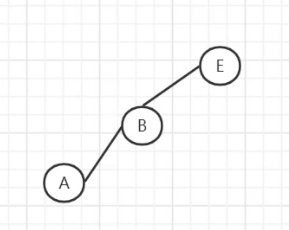现在在左指数上只有C和D没有画出来，

看中根遍历得到：C和D在B的右边，看后根遍历知道D在后面，所以D是根，

在中根遍历中，C在D的左边，所以C是D的左指数；

于是：以E为根节点的左指数画完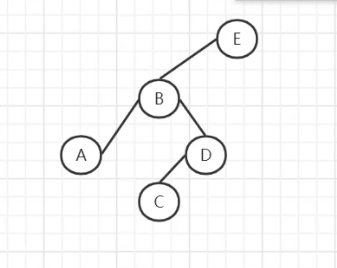接下来看看以E为根节点的右指数，先在后根遍历中找根节点，谁在后面谁是根节点：是F

再到中根遍历中找到F位置，F左边画完了，所以没有左指数，再看右边：GHI

回到后根遍历看GHI，得知H是根节点，所以：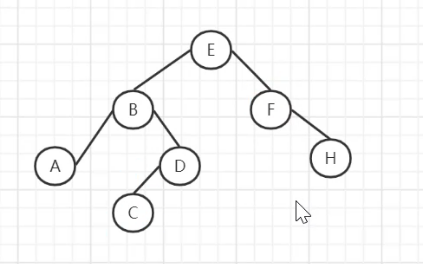现在只剩G、I 没有画了，所以回到中根遍历中看到G在H左，I 在H右，所以：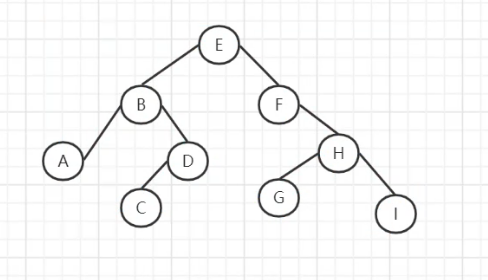如果想知道画的对不对，带进题干，看看他的后根遍历和中根遍历对不对就行了

## 题二：

已知一棵树二叉树的先根遍历和中根遍历的序列分别为:ABDGHCEFI和GDHBAECIF，

请画出此二叉树，并写出它的后根遍的序列

构造的二叉树如下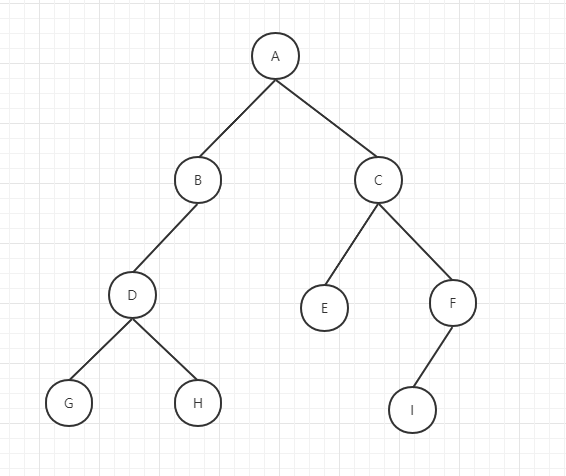### 后根遍历序列: GHDBEIFCA

解题思路：
首先先找根

先根： 根      右                              中根： 左  根

A    BDGH    CEFI                          GDHB   A   ECIF

看先根遍历得知A是根，然后在中根遍历中找到A的位置

所以得知GDHB在A的左边，ECIF在A的右边

那GDHB是谁根呢？回到先根遍历中，谁在前面谁是根，得知是B

再看中根，GDH在B的左边，那就是B的左指数

那GDH种谁是根呢？看先根中，D在前，那D就是根

再去中根遍历中找D的位置，得知左是G，右是H

现在以A为根节点的左指数已全部找到，那右指数就是ECIF

回到先根遍历中，那这四个谁在前面谁是根，是C

再去中根遍历中找C的位置，E在C的左边（左指数），I F在C的右边（右指数）

那 I F谁是根呢？去先根遍历中找：F在前，F是根

中根遍历中 I 在 F 左，于是 I 是F的左指数

展开全文数据结构题 数据结构
• 森林（树）的括号表示法←→森林（树）←→二叉树←→遍历序列 ↓ 遍历序列
• 没区别 如此，中序和后序

没区别如此，中序和后序展开全文• //访问根节点 } } //建立二叉树 //由先根遍历序列和中根遍历序列建立一棵二叉树 public BiTree(String preOrder,String inOrder,int preIndex,int inIndex,int count) { if(count>0) { char r=preOrder....

二叉链式存储结构的结点类描述：

package tree;

public class BiTreeNode {
public Object data;
public BiTreeNode lchild,rchild;  //左右孩子域

//构造一个空节点
public BiTreeNode() {
this(null);
}
//构造一颗左、右孩子域为空的二叉树
public BiTreeNode(Object data) {
this(data,null,null);
}

//构造一棵数据域和左、右孩子域都不为空的二叉树
public BiTreeNode(Object data,BiTreeNode lchild,BiTreeNode rchild) {
this.data=data;
this.lchild=lchild;
this.rchild=rchild;
}
}



二叉链式存储结构下二叉树类的描述：

package tree;
import java.util.Queue;

public class BiTree {
public BiTreeNode root; //树的根节点
public BiTree() {
this.root=null;
}

public BiTree(BiTreeNode root) {
this.root=root;
}

//先根遍历二叉树的递归算法
public void preRootTraverse(BiTreeNode T) {
if(T!=null) {
System.out.print(T.data);  //访问根节点
preRootTraverse(T.lchild);  //先根遍历左子树
preRootTraverse(T.rchild);  //先根遍历右子树
}
}

//中根遍历二叉树的递归算法
public void inRootTraverse(BiTreeNode T) {
if(T!=null) {
inRootTraverse(T.lchild); //中根遍历左子树
System.out.print(T.data);  //访问根节点
inRootTraverse(T.rchild);  //中根遍历右子树
}
}

//后根遍历二叉树的递归算法
public void  postRootTraverse(BiTreeNode T) {
if(T!=null) {
postRootTraverse(T.lchild);  //后根遍历左子树
postRootTraverse(T.rchild);  //后根遍历右子树
System.out.print(T.data);    //访问根节点
}
}
//建立二叉树
//由先根遍历序列和中根遍历序列建立一棵二叉树
public BiTree(String preOrder,String inOrder,int preIndex,int inIndex,int count) {
if(count>0) {
char r=preOrder.charAt(preIndex);
int i=0;
for(;i<count;i++) {
if(r==inOrder.charAt(i+inIndex))
break;
}
root=new BiTreeNode(r);
root.lchild=new BiTree(preOrder,inOrder,preIndex+1,inIndex,i).root;
root.rchild=new BiTree(preOrder,inOrder,preIndex+i+1,inIndex+i+1,count-i-1).root;
}
}
}



测试类：

package tree;

public class test {
public static void main(String[] args) {
// TODO Auto-generated method stub
String preOrder="ABDEGCFH";
String inOrder="DBGEAFHC";
BiTree T=new BiTree(preOrder,inOrder,0,0,preOrder.length());

System.out.println("后根遍历：");
T.postRootTraverse(T.root);
}

}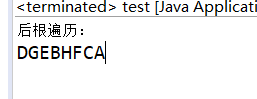展开全文二叉树
• 给定二叉树的2个遍历序列（如先根+中先根+后，中+后等），是否能够根据这2个遍历序列唯一确定二叉树？ 2结论 这三种组合并不是都能唯一确定二叉树的，其中先根+后就不能唯一确定一棵二叉树，中+先根...二叉树 Java
• ## 二叉树的先根遍历

千次阅读 2017-03-03 11:00:28
二叉树的先根遍历面试时，经常问道类似与，获取某一文件夹下所有文件和文件夹；二叉树遍历等问题。 这是一类问题，以二叉树的先根遍历举例：递归实现public class Test { /** * 二叉树节点类 */ public static ...二叉树 递归
• } 创建的二叉树如下图所示 先根遍历:先根遍历就是遍历根节点,然后遍历左子树,再然后右子树 即输出8->4->2->1->3->6->7->12->10->9->11->14->13->15 public void firstRoot() { System.out.print(data + " ");...java 开发语言
• 答： 能确定，树的先根遍历和后根遍历对应二叉树的先序遍历和中序遍历。由二叉树的先序遍历和中序遍历可唯一确定一颗二叉树，二叉树可唯一变换为树
• 那可以推断出，要满足题意的话“二叉树的先序序列与后序序列正好相反”，说明整个二叉树左子树或者右子树有一个没有（遍历就成了，：M-L ；后：L-M 或者 ：M-R ；后：R-M ）也就是必然是一条链。 所以只有A对了...
• 二叉树先根遍历、中根遍历、后根遍历非递归算法的C++代码数据结构 c++ 二叉树
• ## 树的先根遍历

千次阅读 2015-03-28 22:44:14
/*先根遍历兄弟树*/ } } /* void RootFirst(CSTree root) { CSNode *p; if (root!=NULL) { printf("%c ",root->data); / * 访问根结点 * / p= root->FirstChild; while (p!=NULL) { RootFirst(p)...
•二叉树
• 根、中根、后根遍历 三种遍历都是递归遍历 先根遍历 结果：ABCDEFGH 原理：遍历根节点，再遍历左子树，最后遍历右子树； 中根遍历 结果：CBEDFAGH 原理：遍历左子树，再遍历根节点，最后遍历右子树； 后...数据结构 算法
• 递归可以用栈存储，转化为循环 非递归： 递归：
• ## 先根，中根，后根遍历

万次阅读 多人点赞 2018-06-08 16:17:00
先序遍历（根）是访问当前节点，然后再...一棵二叉树的先根遍历为ABCDEFG，中根遍历为CBDEAGF，则其后根遍历为多少？ 方法：（层层解剖，集合从大到小，递归分析） (无论如何必须分析根，确定父节点，再...
• 二叉树先根次序遍历、中次序遍历、后次序遍历Java 二叉树
• 创建一棵二叉树，以二叉链表作存储结构，实现先根遍历算法
• 某二叉树的先根遍历序列和后根遍历序列正好相反，则该二叉树具有的特征是() 正确答案: A 高度等于其结点数 任一结点无左孩子 任一结点无右孩子 空或只有一个结点 添加笔记 ...
• 二叉树的遍历方法有先根遍历、中根遍历、后根遍历以及层次遍历。这里列出了二叉树的根、中根、后根遍历的递归实现以及层次遍历的非递归实现。 我这里用到的是二叉链式结构。话不多说，直接上代码 二叉链式存储结构...二叉树 递归
• // 由中根遍历的数组和后根遍历的数组建立一棵二叉树 // 其中参数inOrder是整棵树的中根遍历，postOrder是整棵树的后根遍历，inIndex是 // 中根遍历从inOrder字符串中的开始位置，postIndex是后根遍历从字符串...
• ## 二叉树先根、中根、后根遍历

万次阅读 多人点赞 2017-10-14 17:39:19
二叉树根、中根、后根遍历 先根遍历: ABCDEFGH 中根遍历：CBEDFAGH 后根遍历 : CEFDBHGA
• 二叉树有三种遍历方式：先序遍历，中序遍历，后续遍历 即：中后指的是访问节点的顺序 eg:先序 左右 中序 左右 后序 左右 遍历总体思路：将树分成最小的子树，然后按照顺序输出 1.1 先序遍历 　a 访问...
• 函数填空：层次遍历多元树（在文件tree.cpp中3个空）、先根遍历、后根遍历的递归函数（在文件tree.h中2个空）；
• 二叉树( Binary Tree) 是 n个结点的有限集合，该集合或者为空集(称为空二叉树)，戴者由一个结点和两颗互不相交的、分别称为结点的左子树和右子树的二叉树组成 。 二叉树结点的定义: typed数据结构 二叉树
• 通过比较二叉树的先根遍历，中根遍历，后根遍历的非递归算法可以发现：这三个算法的实现是极其相似的（如同它们递归算法的也很相似一般）。 1：都用到了栈来暂存节点。 2：都是两个while的嵌套循环。 ...iostream struct Struct 九度oj 存储
• //由根和中根遍历建立二叉树 public class bitree{  public bitree(String preorder,String inorder,int preindex,int inindex,int count){  if(count>0){ //根中根为空  char r=preorder.char...